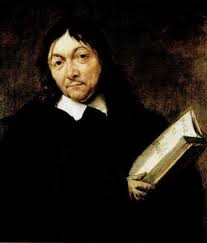• 1596 March 31
(b.) -
1650 February 11
(d.)

#### Bio/Description

A French philosopher and writer who spent most of his adult life in the Dutch Republic, his influence in mathematics is equally apparent; the Cartesian coordinate system allowing algebraic equations to be expressed as geometric shapes (2D coordinate system) was named after him. He is credited as the father of analytical geometry the bridge between algebra and geometry, crucial to the discovery of infinitesimal calculus and analysis. This revolutionized mathematics by providing the first systematic link between Euclidean geometry and algebra. Using the Cartesian coordinate system, geometric shapes (such as curves) can be described by Cartesian equations: algebraic equations involving the coordinates of the points lying on the shape. Cartesian coordinates are the foundation of analytic geometry, and provide enlightening geometric interpretations for many other branches of mathematics, such as linear algebra, complex analysis, differential geometry, multivariate calculus, group theory, and more. A familiar example is the concept of the graph of a function. Cartesian coordinates are also essential tools for most applied disciplines that deal with geometry, including astronomy, physics, engineering, and many more. They are the most common coordinate system used in computer graphics, computer-aided geometric design, and other geometry-related data processing. He was also one of the key figures in the Scientific Revolution.
• Date of Birth:

1596 March 31
• Date of Death:

1650 February 11
• Gender:

Male
• Noted For:

He is credited as the father of analytical geometry the bridge between algebra and geometry
• Category of Achievement: